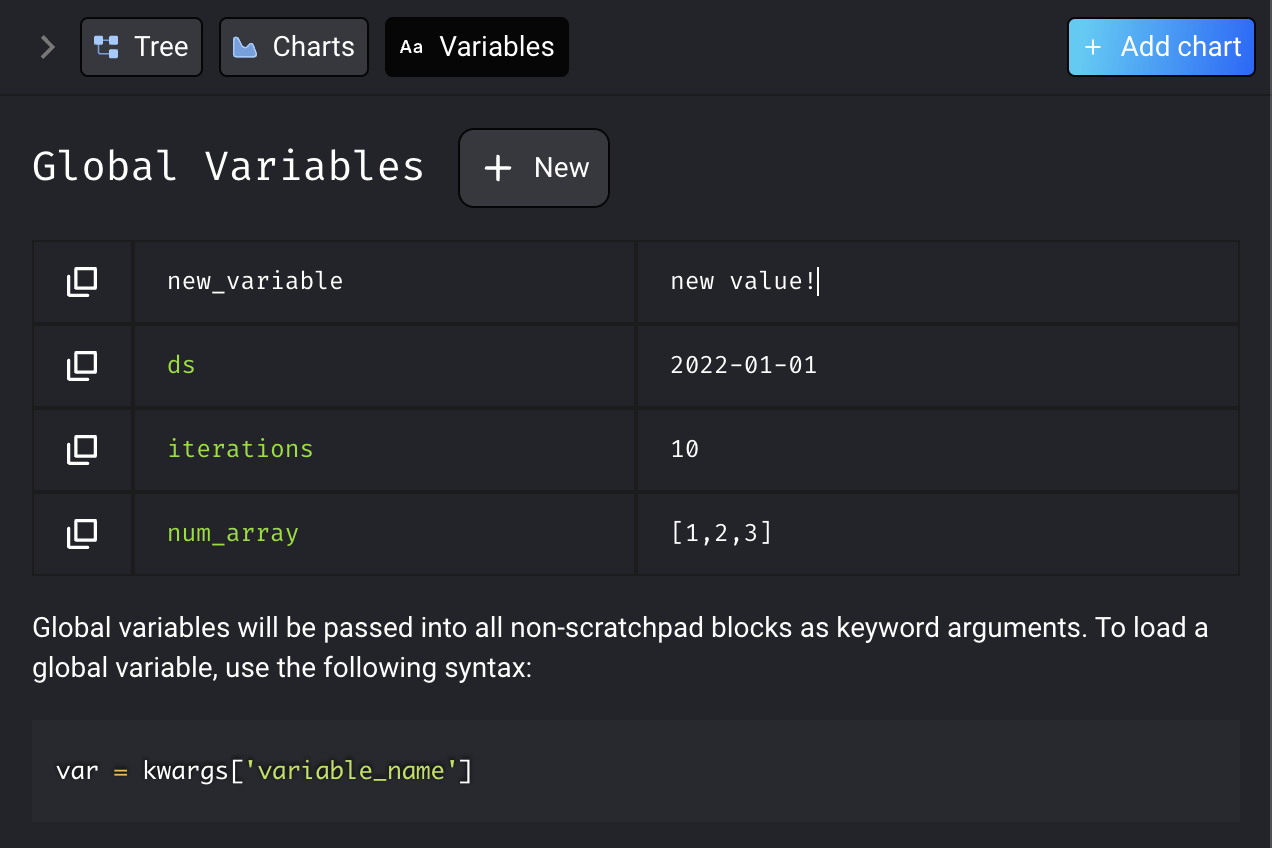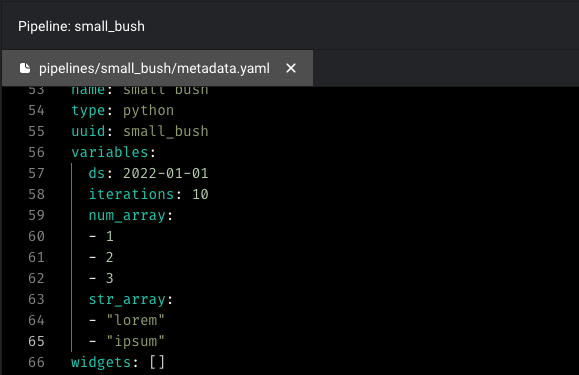Runtime Variables are a set of global variables that can be used by every block. These are useful for storing constants shared by multiple blocks or constants whose value is determined at pipeline runtime (hence runtime variables).

## ​Using Runtime Variables

Runtime Variables can be accessed via the `**kwargs` parameter in your block function.

``````from pandas import DataFrame
from os import path

filepath = kwargs.get('filepath')
row_limit = kwargs.get('row_limit')
``````

Currently, runtime variables must be of primitive Python types or some basic containers:

• integer
• string
• float
• boolean
• list
• dictionary
• set

Runtime variable names must be a valid Python identifier (i.e., no spaces in name, can’t start with a number).

## ​Creating Runtime Variables

### ​In Mage editor

You can create new global variables from the Mage UI through the “Variables” tab in the sidekick. Click the “New” button, and configure your variable’s name and value, and press Enter to save.

To edit global variables, hover over the variable and click on the edit button. You can edit the variable name and/or value, and press Enter to save.### ​In code

You can also create or edit global variables in code by editing the pipeline `metadata.yaml` file.### ​In Python code

You can also save the variable in Python code. One example usage is to save checkpoint data.

``````from mage_ai.data_preparation.variable_manager import set_global_variable
set_global_variable(pipeline_uuid, key, value)
``````

## ​Default Runtime Variables

Mage provides some default variables to give context on the pipeline execution.

• `execution_date`: A datetime object that the pipeline is executed at.
• `event`: If the pipeline is triggered by event, the `event` variable contains the event payload.

## ​Running Pipeline with Runtime Variables

### ​Run from command line

You can execute your pipeline with runtime variables from the command line. First, make sure you installed the package.

``````pip install mage-ai
``````

Once the package is installed, you can run your pipeline through the command line.

``````mage run <project_path> <pipeline_uuid> --runtime-vars [name value]...

# example with 2 runtime variables "name" and "ds":
mage run default_repo default_pipeline --runtime-vars name default ds 2022-08-18
``````

### ​Run from Python script

If your pipeline is configured to use runtime variables, you can still execute your pipeline outside the code editor. Provide the runtime variables as keyword arguments to `mage_ai.run()`:

``````mage_ai.run('sample_pipeline', 'repos/default_repo', filepath = 'path/to/my/file.csv', row_limit=1000)
``````

## ​Example - Aggregating Daily Logs

A common use of ETL pipelines is to process and analyze daily events. In the case of this example, we will create an ETL pipeline to analyze log messages from a web application. Suppose the following is an example of a log file that our web application produces.

log_datetypesource
2022-07-27T20:19:20INFOreact-1
2022-07-27T19:18:45WARNINGexpress-2
2022-07-27T16:35:28DEBUGreact-1
2022-07-27T10:19:32INFOexpress-1
2022-07-27T07:20:26ERRORexpress-1
2022-07-27T00:42:37ERRORreact-1

Suppose we want to know the distribution of log types at the end of every day. Using Mage’s runtime variables this is made a very simple task:

1. Create a data loader to load all log files modified on a specific date. We will specify the log folder and the date to load logs from using runtime variables which are passed to this block via the `**kwargs` parameter.

``````from datetime import datetime, timedelta, date
from pandas import DataFrame, concat, read_csv
from pathlib import Path
import os

start = datetime.fromisoformat(kwargs.get('current_date'))
end = start + timedelta(days=1)
logpath = Path(kwargs.get('log_folder'))
logs = []
for file in logpath.iterdir():
print(file)
modification_time = datetime.fromtimestamp(os.path.getmtime(file))
if modification_time >= start and modification_time < end:
logs.append(df)
return concat(logs, axis=0)
``````

Note: This code ignores the edge case of a log file that spills over between days. Since the modification date is used instead of the creation date, a log file that is modified between days will only be considered in the latter day.

2. Calculate the distribution of log types over this date

``````from pandas import DataFrame
from os import path
import pandas as pd

if 'transformer' not in globals():
from mage_ai.data_preparation.decorators import transformer

@transformer
def extract_statistics(df: DataFrame, **kwargs) -> DataFrame:
now = kwargs.get('current_date')
count = df['type'].value_counts()
count = DataFrame({now: count}).T
return count
``````

The result of this transformer is a new data frame that looks like below:

DEBUGERRORINFOWARNING
2022-07-28816765828871

This data can then be ingested into a log statistics database.

Key: As the current date and log folder are not hardcoded in the pipeline but instead provided as a runtime variable, your pipeline code remains reusable without having to change any code. Every day, the pipeline can be ran using `mage_ai.run()`, providing the current date and log folder as keyword arguments:

``````mage_ai.run('log_stats_ingestion', 'repos/default_repo', current_date='2022-07-29', log_folder='logs/webapp')
``````

## ​Example - Model Rockets

Consider the following sample data tracking the launch angle (in degrees) and vertical velocity (in meters per second) of model rocket tests:

launch_idanglevertical_velocity
128.461.34
214.432.03
361.4113.19
444.289.96
539.582.00
679.3126.73
774.9124.51
838.179.6

Suppose we want to convert the launch angle from degrees to radians in our pipeline. The example below uses the `pi` runtime variable (passed in through `**kwargs`) to convert the degrees column of some input data frame to a radians. This allows us to control the precision with which we want to store `pi`.

``````from pandas import DataFrame
from os import path

if 'transformer' not in globals():
from mage_ai.data_preparation.decorators import transformer

@transformer
def convert_to_radians(df: DataFrame, **kwargs) -> DataFrame:
pi = kwargs.get('pi')
df['angle_radians'] = df['angle'] * pi / 180
return df
``````

Suppose also want to convert the velocity from meters per second to kilometers per hour by multiplying by the constant 3.6. Using the runtime variable `conversion_factor` (again passed in through `**kwargs`), the vertical velocity in kilometers per hour can be computed:

``````from pandas import DataFrame
from os import path

if 'transformer' not in globals():
from mage_ai.data_preparation.decorators import transformer

@transformer
def convert_velocity(df: DataFrame, **kwargs) -> DataFrame:
conversion_factor = kwargs.get('conversion_factor')
df['converted_vertical_velocity'] = df['vertical_velocity'] * conversion_factor
return df
``````

Now that your pipeline is complete, you can run your pipeline using `mage_ai.run()`. Specify values for runtime variables as keyword arguments to this function:

``````mage_ai.run('model_rocket_data_ingestion', 'repos/default_repo', pi=3.1415, conversion_factor=3.6)
``````

Suppose after creating your pipeline, you instead want to store your velocity in miles per hour instead of kilometers per hour. As the conversion factor is a runtime variable, you don’t have to edit your pipeline - you just have to change the conversion factor which your pipeline is run with!

``````mage_ai.run('model_rocket_data_ingestion', 'repos/default_repo', pi=3.1415, conversion_factor=2.24)
``````